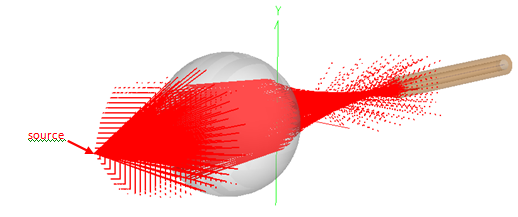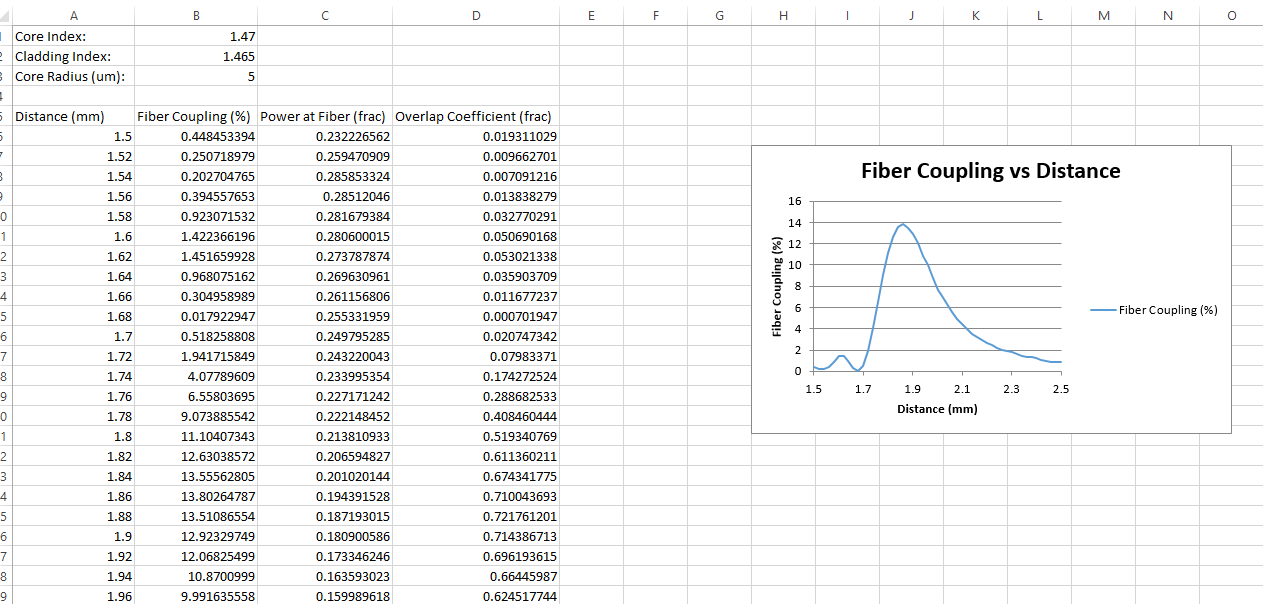# Outputting data to Microsoft Excel

The example file associated with the Laser Diode to Fiber article includes Embedded Scripts that scan the position/orientation of the fiber and automatically output the data to Microsoft Excel using FRED's COM capability.There are three Embedded Scripts associated with the attached FRED document:

•          Distance Scan
•          Lateral Offset Scan
•          Tilt Scan

The three scripts are similar to each other: each script adjusts the position of the fiber in user-controlled steps and calculates the coupling efficiency and prints the data to the Output Window and into a Microsoft Excel spreadsheet.

Steps

First, input the start and end positions of the fiber and the resolution of the scan. Then, set the exportToExcel flag to True to print the data to a Microsoft Excel spreadsheet and plot a graph. Define the fiber parameters for the fiber coupling efficiency calculation only.

```    '***********SCAN SETTINGS************
'Z-position of the fiber interface
startVal    = 1.5
endVal      = 2.5
resolution  = 0.02
exportToExcel = True
'***********SCAN SETTINGS************<br>
```

Before outputting data to Excel, create the Excel Application object ("excelApp"), add a workbook ("excelWB"), and a starting cell location ("excelRange").

```    If exportToExcel = True Then
'set up Excel
Set excelApp = CreateObject("Excel.Application")
Set excelRange = excelWB.ActiveSheet.Cells(1,1)
excelApp.Visible = True

'print fiber details and headers to Excel
excelRange.Cells(1,1).Value = "Core Index: "
excelRange.Cells(1,2).Value = n_core
excelRange.Cells(3,1).Value = "Core Radius (um): "
excelRange.Cells(3,2).Value = r*1000

excelRange.Cells(5,1).Value = "Distance (mm)"
excelRange.Cells(5,2).Value = "Fiber Coupling (%)"
excelRange.Cells(5,3).Value = "Power at Fiber (frac)"
excelRange.Cells(5,4).Value = "Overlap Coefficient (frac)"

cellpos = 5     'Excel row counter
End If<br>
```

The script prints the headers and starts its main "For" loop which:

• alters the position of the fiber
• traces the rays
• calculates the Irradiance & determine the total power
• calculates the fiber coupling coefficient
• calculates the mode power

The script then writes the data to both the Output Window and to Excel. Notice that the cell position is specified for each input.

```        'print results to Excel
If exportToExcel = True Then
cellpos = cellpos + 1
excelRange.Cells(cellpos,1).Value = i
excelRange.Cells(cellpos,2).Value = modePower * 100
excelRange.Cells(cellpos,3).Value = fiberPower
excelRange.Cells(cellpos,4).Value = CE
End If<br>
```

After the simulations are complete, FRED instructs Excel to plot a graph of the data using the following code. The result is shown below (in Excel 2013).

```        'Now create graph in Excel
excelChart.ChartType = 75 'scatter plot
excelChart.HasTitle = True
excelChart.name = "Fiber Coupling vs Distance"
excelChart.ChartTitle.text = "Fiber Coupling vs Distance"
excelChart.SetSourceData(excelRange.Range("a5:b"&CStr(cellpos)))
excelChart.PlotBy = 2 'plots by columns.
excelChart.Axes(1).MinimumScale = startVal
excelChart.Axes(1).MaximumScale = endVal
excelChart.Axes(1).HasTitle = True
excelChart.Axes(1).AxisTitle.text = "Distance (mm)"
excelChart.Axes(2).HasTitle = True
excelChart.Axes(2).AxisTitle.text = "Fiber Coupling (%)"
excelChart.Location 2, "Sheet1"<br>
```The final step is to close the Excel application and clean up the variables.

```        'Excel clean up
Set excelRange = Nothing
Set excelWB = Nothing
Set excelApp = Nothing
```

Associated FRED file:  Laser Diode to Fiber Coupling Analysis.frd